×#### Thank you for registering.

One of our academic counsellors will contact you within 1 working day.

Click to Chat

1800-1023-196

+91-120-4616500

CART 0

• 0

MY CART (5)

Use Coupon: CART20 and get 20% off on all online Study Material

ITEM
DETAILS
MRP
DISCOUNT
FINAL PRICE
Total Price: Rs.

There are no items in this cart.
Continue Shopping• Complete JEE Main/Advanced Course and Test Series
• OFFERED PRICE: Rs. 15,900
• View Details

```Chapter 24: Measures of Central Tendency Exercise – 24.1

Question: 1

If the heights of 5 persons are 140 cm, 150 cm, 152 cm, 158 cm and 161 cm respectively. Find the mean height.

Solution:

Given: The heights of 5 persons are 140 cm, 150 cm, 152 cm, 158 cm and 161 cmQuestion: 2

Find the mean of 994, 996, 998, 1000, 1002.

Solution:

Numbers are 994, 996, 998, 1000, 1002.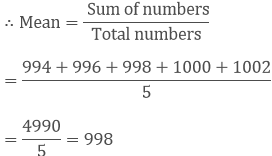Mean = 998

Question: 3

Find the mean of first five natural numbers.

Solution:

The first five odd numbers are 1, 2, 3, 4, 5.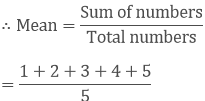= 15/5 = 3

Mean = 3

Question: 4

Find the mean of all factors of 10.

Solution:

All factors of 6 are 1, 2, 5, 10.Mean = 4.5

Question: 5

Find the mean of first ten even natural numbers.

Solution:

The first five even natural numbers are 2 , 4 , 6 , 8 , 10 , 12 , 14 , 16 , 18 , 20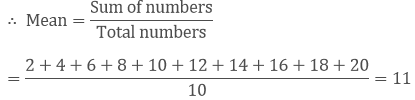Mean = 11

Question: 6

Find the mean of x, x + 2, x + 4, x + 6, x + 8.

Solution:

Numbers are x, x + 2, x + 4, x + 6, x + 8.= x + 4

Question: 7

Find the mean of first five multiples of 3.

Solution:

First five multiples of 3 are 3, 6, 9, 12, 15.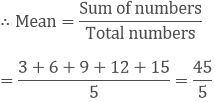= 9

Mean = 9

Question: 8

Following are the weights of 10 new born babies in a hospital on a particular day: 3.4 , 3 .6 , 4.2 , 4.5 , 3.9 , 4.1 , 3.8 , 4.5 , 4.4 , 3.6 (in kg). Find the mean.

Solution:

The weights (in kg) of 10 new born  babies are : 3.4 , 3 .6 , 4.2 , 4.5 , 3.9 , 4.1 , 3.8 , 4.5 , 4.4 , 3.6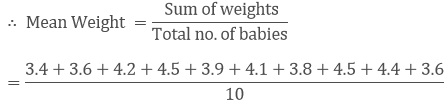= 4 kg

Question: 9

The percentage marks obtained by students of a class in mathematics are as follows: 64, 36 , 47, 23, 0, 19, 81, 93, 72, 35, 3, 1 .Find their mean.

Solution:

The percentage marks obtained by students are 64, 36 , 47, 23, 0, 19, 81, 93, 72, 35, 3, 1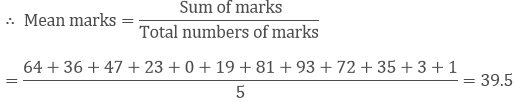Mean Marks = 39.5

Question: 10

The numbers of children in 10 families of a locality  are 2, 4, 3, 4, 2, 3, 5, 1, 1, 5. Find the number of children per family.

Solution:

The numbers of children  in 10 families  are : 2, 4, 3, 4, 2, 3, 5, 1, 1, 5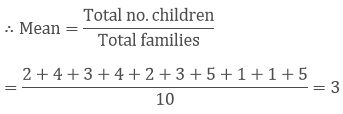Question: 11

If M is the mean of x1, x2,x3,x4,x5 and x6, Prove that (x1 − M) + (x2 − M) + (x3 − M) + (x4 − M) + (x5 − M) + (x6 − M) = 0.

Solution:

Let M be the mean of x1, x2, x3, x4, x5 and x6

Then,= x1 + x2 + x3 + x4 + x5 + x6 = 6M

To Prove :- (x1 − M) + (x2 − M) + (x3 − M) + (x4 − M) + (x5 − M) + (x6 − M) = 0.

Proof: L. H. S

= (x1 − M) + (x2 − M) + (x3 − M) + (x4 − M) + (x5 − M) + (x6 − M)

= (x1 + x2 + x3 + x4 + x5 + x6) − (M + M + M + M + M + M)

= 6M – 6M

= 0

= R.H.S

Question: 12

Duration of sunshine(in hours) in  Amritsar  for first  10 days of August  1997 as reported by the Meterological Department  are given as  follows : 9.6 , 5.2 , 3.5 , 1.5 , 1.6 , 2.4 , 2.6 , 8.4 , 10.3 , 10.9

1. Find the mean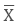2. Verify that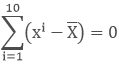Solution:

Duration of sunshine (in hours ) for 10 days are = 9.6 , 5.2 , 3.5 , 1.5 , 1.6 , 2.4 , 2.6 , 8.4 , 10.3 , 10.9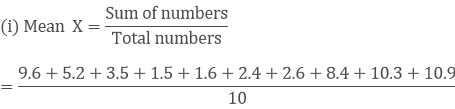= 56/10 = 5.6

(ii) L.H.S == (9.6 − 5.6) + (5.2 − 5.6) + (3.5 − 5.6) + (1.5 − 5.6) + (1.6 − 5.6) + (2.4 − 5.6) + (2.6 − 5.6) + (8.4 − 5.6) + (10.3 − 5.6) + (10.9 − 5.6)

= 4 – 0.4 – 2.1 – 4.1 – 4 – 3.2 – 3 + 2.8 + 4.7 + 5.3

= 16.8 – 16.8 = 0

= R.H.S

Question: 13

Explain, by taking a suitable example, how the arithmetic mean alters by (i) adding a constant k to each term, (ii) Subtracting a constant k from each term, (iii) multiplying each term by a constant k and (iv) dividing each term by non-zero constant k.

Solution:

Let say numbers are 3, 4, 5(i). Adding constant term k = 2 in each term.

New numbers are = 5, 6, 7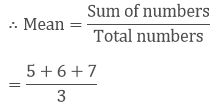∴ new mean will be 2 more than the original mean.

(ii). Subtracting constant term k = 2 in each term.

New numbers are = 1, 2, 3∴ new  mean will be 2 less than the original mean.

(iii) . Multiplying by constant term k = 2 in each term.

New numbers are = 6, 8, 10= 8 = 4 × 2

∴ new  mean will be 2 times of  the original mean.

(iv) . Divide the constant term k = 2 in each term.

New numbers are = 1.5, 2, 2.5.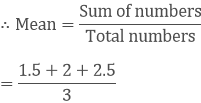= 2 = 4/2

∴ new mean will be half  of  the original mean.

Question: 14

The mean of marks scored by 100 students was found to be 40. Later on, it was discovered that a score of 53 was misread as 83. Find the correct mean.

Solution:

Mean marks of 100 students = 40

Sum of marks of 100 students = 100 × 40

= 4000

Correct value = 53

Incorrect value = 83

Correct sum = 4000 – 83 + 53 = 3970

∴ correct mean = 3970/100 = 39.7

Question: 15

The traffic police recorded the speed (in km/hr) of 10 motorists as 47 , 53 , 49 , 60 , 39 , 42 , 55 , 57 , 52 , 48 . Later on, an error in recording instrument was found. Find the correct average speed of the motorists if the instrument is recorded 5 km/hr less in each case.

Solution:

The speed of 10 motorists are 47 , 53 , 49 , 60 , 39 , 42 , 55 , 57 , 52 , 48 .

Later on it was discovered that the instrument recorded 5 km/hr less than in each case

∴ correct values are = 52 , 58 , 54 , 65 , 44 , 47 , 60 , 62 , 57 , 53.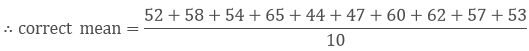= 552/10 = 55.2 km/hr

Question: 16

The mean of five numbers is 27. If one number is excluded, their mean is 25. Find the excluded number.

Solution:

The mean of five numbers is 27

The sum of five numbers = 5 × 27 = 135

If one number is excluded, the new mean is 25

∴ Sum of 4 numbers = 4 × 25 = 100

∴ Excluded number = 135 – 100 = 35

Question: 17

The mean weight per student in a group of 7 students is 55 kg. The individual weights of 6 of them (in kg) are 52, 54, 55, 53, 56 and 54. Find the weight of the seventh student.

Solution:

The mean weight per student in a group of 7 students = 55 kg

Weight of 6 students (in kg) = 52, 54, 55, 53, 56 and 54

Let the weight of seventh student = x kg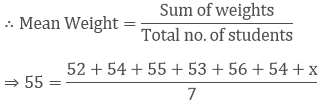⇒ 385 = 324 + x

⇒ x = 385 − 324

⇒ x = 61 kg

∴ weight of seventh student = 61 kg.

Question: 18

The mean weight of 8 numbers is 15. If each number is multiplied by 2, what will be the new mean?

Solution:

We have,

The mean weight of 8 numbers is 15

Then, the sum of 8 numbers = 8 × 15 = 120

If each number is multiplied by 2

Then, new mean = 120 × 2 = 240

∴ new mean = 240/8 = 30.

Question: 19

The mean of 5 numbers is 18. If one number is excluded, their mean is 16. Find the excluded number.

Solution:

The mean of 5 numbers is 18

Then, the sum of 5 numbers = 5 × 18 = 90

If one number is excluded

Then, the mean of 4 numbers = 16

∴ sum of 4 numbers = 4 × 16 = 64

Excluded number = 90 – 64 = 26.

Question: 20

The mean of 200 items was 50. Later on, it was on discovered that the two items were misread as 92 and 8 instead of 192 and 88. Find the correct mean.

Solution:

The mean of 200 items = 50

Then the sum of 200 items = 200 × 50 = 10,000

Correct values = 192 and 88.

Incorrect values = 92 and 8.

∴ correct sum = 10000 – 92 – 8 + 192 + 88 = 10180

∴ correct mean = 10180/200 = 101.8/2 = 50.9 .

Question: 21

Find the values of n andin each of the following cases: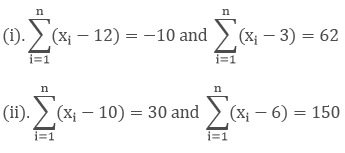Solution:⇒ (x1 − 12) + (x2 − 12) + ……… + (xn − 12) = −10

⇒ (x1 + x2 + x3 + x4 + x5 + ⋅⋅⋅ +xn) − (12 + 12 + 12 + 12 + ⋅⋅⋅⋅ + 12) = −10

⇒ ∑x − 12n = −10 ⋅⋅⋅⋅ (1)⇒ (x1 − 3) + (x2 − 3) + ⋅⋅⋅⋅ + (xn − 3) = 62

⇒ (x1 + x2 + ⋅⋅⋅ + xn) − (3 + 3 + 3 + ⋅⋅⋅ + 3) = 62

⇒∑ x − 3n = 62 ⋅⋅⋅⋅⋅ (2)

By subtracting equation (1) from equation (2), we get

∑x − 3n − ∑x + 12n = 62 + 10

⇒ 9n = 72

⇒ n = 72/9 = 8

Put value of n in equation (1)

∑x − 12 × 8 = −10

⇒ ∑x − 96 = −10

⇒∑x = 96 − 10 = 86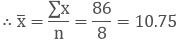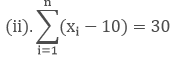(x1 − 10) + (x2 − 10) +  ………… + (xn − 10) = 30

⇒ (x1 + x2 + x3 + x4 + x5 + ⋅⋅⋅ + xn) − (10 + 10 + 10 + 10 + ⋅⋅⋅⋅ + 10) = 30

⇒ ∑x − 10n = 30 ⋅⋅⋅⋅ (1)⇒ (x1 − 6) + (x2 − 6) + ⋅⋅⋅⋅⋅ + (xn − 6) = 150

⇒ (x1 + x2 + ⋅⋅⋅ + xn) − (6 + 6 + 6 + ⋅⋅⋅ + 6) = 150

⇒∑x − 6n = 150 ⋅⋅⋅⋅ (2)

By subtracting equation (1) from equation (2), we get

∑x − 6n − ∑x + 10n = 150 − 30

⇒ 4n = 120

⇒ n = 120/4 = 30

Put value of n in equation (1)

∑x − 10 × 30 = 30

⇒ ∑x − 300 = 30

⇒ ∑x = 30 + 300 = 330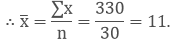Question: 22

The sum of the deviations of a set of n values x1, x2, x3,⋅⋅⋅, xn measured from 15 and -3 are -90 and 54 respectively . Find the value of n and mean.

Solution:

Given: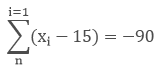⇒ (x1 − 15) + (x2 − 15) + ⋅⋅⋅⋅⋅ + (xn − 15) = −90

⇒ (x1 + x2 + ⋅⋅⋅⋅ + n) − (15 + 15 + 15+ ⋅⋅⋅⋅⋅⋅ + 15) = −90

⇒ ∑x − 15n = −90 ⋅⋅⋅⋅ (1)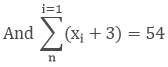⇒ (x1 + 3) + (x2 + 3) + ⋅⋅⋅⋅⋅ + (xn + 3) = 54

⇒ (x1 + x2 + ⋅⋅⋅⋅ + n) + (3 + 3 + 3 + ⋅⋅⋅⋅⋅⋅ + 3) = 54

⇒ ∑x + 3n = 54 ⋅⋅⋅ (2)

By subtracting equation (1) from equation (2), we get

∑x + 3n − ∑x + 15n = 54 + 90

⇒18n=144

⇒ n = 144/18 = 8

Put value of n in equation (1)

∑x − 15 × 8 = − 90

∑x − 120 = − 90

∑x = 120 − 90 = 30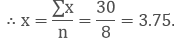Question: 23

Find the sum of the deviations of the variate values 3, 4, 6, 7, 8, 14 from their mean.

Solution:

Values 3, 4, 6, 7, 8, 14∴ Mean = 42/6

= 7

∴ Sum of deviation of values from their mean

= (3 − 7) + (4 − 7) + (6 − 7) + (7 − 7) + (8 − 7) + (14 − 7)

= – 4 – 3 – 1 + 0 + 1 + 7

= – 8 + 8 = 0

Question: 24

Ifis the mean of the ten natural numbers x1, x2, x3, ⋅⋅⋅, x10 show that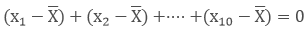Solution:

We have,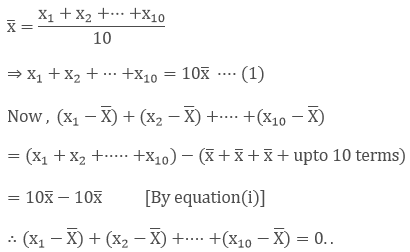```### Course Features

• 728 Video Lectures
• Revision Notes
• Previous Year Papers
• Mind Map
• Study Planner
• NCERT Solutions
• Discussion Forum
• Test paper with Video Solution